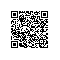# DP之矩阵连乘问题

a，铺垫的数学知识首先要搞清楚矩阵相乘是怎么乘的：

1）对于连续的n个矩阵相乘 A1 * A2 *A3.........An，其乘法顺序可以是任意的，可以在上面加括号，改变做乘法的顺序，例如 A*B*C三个矩阵相乘可以A*(B*C)

2）对于2个m*p，p*n的矩阵相乘，共做乘法次数为 m*n*p 次。这是预备知识，知道矩阵连续的乘法的运算次数跟运算顺序有关后，就很容易举出例子了，略。
b，卡特兰数个，证明很麻烦，有时间看了组合数学再来看
c，重点是要解决这个问题。

Ai *.......Ak * Ak+1.....Aj

M[i , j]  =  min{ M[i , k] + M[k+1 , j]  + p[i - 1] * p[ k ] * p[ j ] }         i   <=   k   <=   j-1

package Section8;

/*第八章 动态规划   课后习题：矩阵连乘*/

public class MatEven {

/**
* @param args
*/
public static void main(String[] args) {
// TODO Auto-generated method stub
int[] Dim = {30,35,35,15,15,5,5,10,10,20,20,25};
int result = MatEven(Dim);

System.out.println("\n动态规划求的的最优策略相乘顺序导致的最少乘法数为：" + result);
}

public static int MatEven(int[] Dim){
//接受n个矩阵的维度数组Dim大小为2n
int n = Dim.length / 2;        //有n个矩阵，编号0...n-1,编号为k的矩阵的维数是Dim[2k] * Dim[2k+1]

int[][] Result = new int[n][n];        //最小代价矩阵

//初始化
for(int i = 0;i < n;i++)
Result[i][i] = 0;

//沿对角线填矩阵
for(int d = 1;d <= n-1;d++)      //共n-1条对角线需要填
{
for(int i = 0;i <= n-d-1;i++)    //第d条对角线的第一个点横坐标为d
{
//int j = i - d;
int j = i + d;        //在第d条对角线上的点，横纵坐标的关系是j = i + d

//这样就确定了一个位置（i,j）的坐标，然后来填（i,j）
int Min = 1000000000;
for(int k = i;k <= j-1;k++)        //从第k个矩阵后面断开
{
//动态规划状态转移方程
int temp = Result[i][k] + Result[k+1][j] + (Dim[2*i] * Dim[2*k + 1] * Dim[2*j+1]);
if( temp < Min)
Min = temp;
}

Result[i][j] = Min;
}

}

return Result[n-1];
}
}

-------------------------------------------------------------------------

----------------------------------------------------------------------------------------------使用钉钉扫一扫加入圈子
+ 订阅